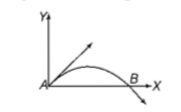If vectors A=cosωt $\stackrel{^}{i}$+sinωt $\stackrel{^}{j}$ and B=cosωt/2$\stackrel{^}{i}$+sinωt/2$\stackrel{^}{j}$ are functions of time, then the value of t at which they are orthogonal to each other

1. t=π/4ω

2. t=π/2ω

3. t=π/ω

4. t=0

Concept Questions :-

Position and displacement
High Yielding Test Series + Question Bank - NEET 2020

Difficulty Level:

The position vector of a particle  as a function of time is given by r = 4 sin (2πt) $\stackrel{^}{i}$+ 4 cos (2πt) $\stackrel{^}{j}$ where r is in metre, t is in seconds, $\stackrel{^}{i}$ and $\stackrel{^}{j}$ denote unit vectors along x and y-directions, respectively. Which one of the following statements is wrong for the motion of particle?

1. Acceleration is along-R

2.  Magnitude of acceleration vector is v2/R where v is the velocity of particle

3.  Magnitude of the velocity of particle is 8 m/s

4.  Path of the particle is a circle of radius 4 m

Concept Questions :-

Circular motion
High Yielding Test Series + Question Bank - NEET 2020

Difficulty Level:

Concept Questions :-

Projectile motion
High Yielding Test Series + Question Bank - NEET 2020

Difficulty Level:

A particle is moving such that its position coordinates (x, y) are (2m, 3m) at time t=0, (6m, 7m) at time t=2s and (13m, 14m) at time t=5s. Average velocity vector (vav) from t=0 to t=5s is

1. $\frac{1}{5}$(13$\stackrel{^}{i}$+14$\stackrel{^}{j}$)

2. $\frac{7}{3}$($\stackrel{^}{i}$+$\stackrel{^}{j}$)

3. 2($\stackrel{^}{i}$+$\stackrel{^}{j}$)

4. $\frac{11}{5}$($\stackrel{^}{i}$+$\stackrel{^}{j}$)

Concept Questions :-

Speed and velocity
High Yielding Test Series + Question Bank - NEET 2020

Difficulty Level:

The velocity of a projectile at the initial point A is (2i+3j) m/s. Its velocity (in m/s) at point B is:1.  -2i+3j

2.  -2i-3j

3.   2i-3j

4.  2i+3j

Concept Questions :-

Projectile motion
High Yielding Test Series + Question Bank - NEET 2020

Difficulty Level:

The horizontal range and the maximum height of a projectile are equal.The angle of projection of the projectile is

1. $\theta ={\mathrm{tan}}^{-1}\left(\frac{1}{4}\right)$                                       2. $\theta ={\mathrm{tan}}^{-1}\left(4\right)$

3. $\theta ={\mathrm{tan}}^{-1}\left(2\right)$                                          4. $\theta =45°$

Concept Questions :-

Projectile motion
High Yielding Test Series + Question Bank - NEET 2020

Difficulty Level:

A particle has initial velocity (2i + 3j) and acceleration (0.3i + 0.2j). The magnitude of velocity after 10s will be

1. $9\sqrt{2}units$                                   2. $5\sqrt{2}units$

3. 5units                                         4. 9units

Concept Questions :-

Uniformly accelerated motion
High Yielding Test Series + Question Bank - NEET 2020

Difficulty Level:

A body is moving with velocity 30 m/s towards east. After 10 s its velocity becomes 40 m/s towards north. The average acceleration of the body is

(a)                                              (b)

(c)                                              (d)

Concept Questions :-

Acceleration
High Yielding Test Series + Question Bank - NEET 2020

Difficulty Level:

A missile is fired for maximum range with an initial velocity of 20 m/s. If g=10 $\mathrm{m}/{\mathrm{s}}^{2}$, the range of the missile is

1. 50 m                                                          2. 60 m

3. 20 m                                                          4. 40 m

Concept Questions :-

Projectile motion
High Yielding Test Series + Question Bank - NEET 2020

Difficulty Level:

A projectile is fired at an angle of 45$°$ with the horizontal. The elevation angle of the projectile at its highest point as seen from the point of projection is:

1. 60$°$                                           2. ${\mathrm{tan}}^{-1}\left(\frac{1}{2}\right)$

3. ${\mathrm{tan}}^{-1}\left(\frac{\sqrt{3}}{2}\right)$                               4. $45°$

Concept Questions :-

Projectile motion